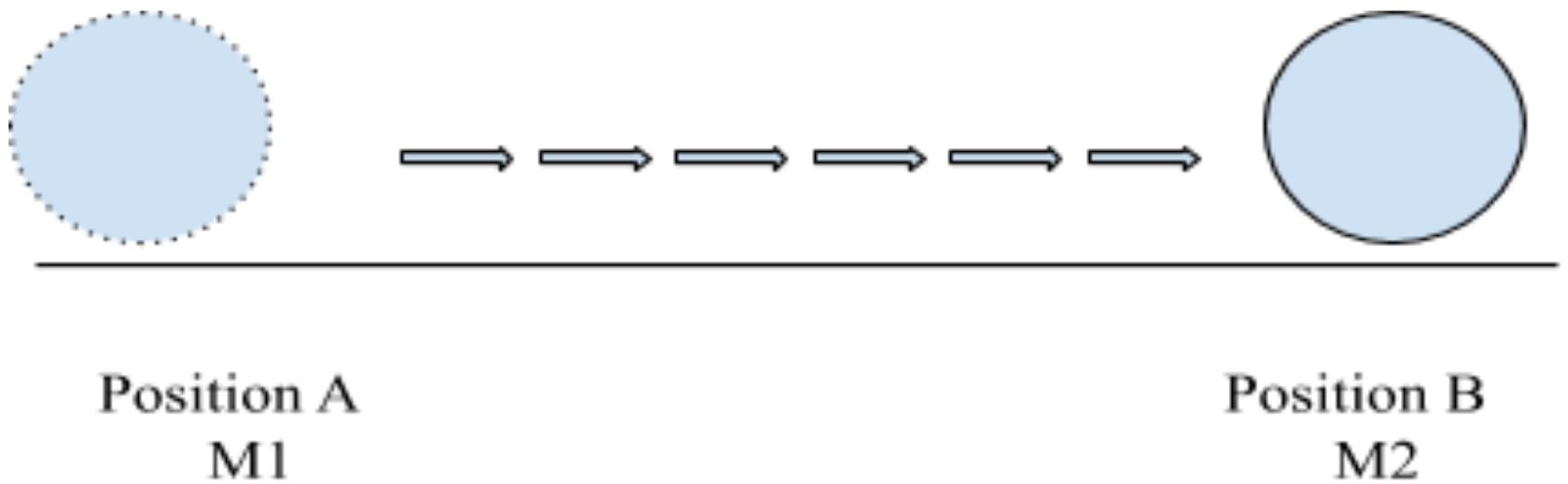# Newton’s second law of motion

Author: ARAS

Newton’s second law is the quantitative definition of the law of inertia i.e Newton’s first law. It basically deals with the momentum of the body which is mass into velocity.  In mathematical form is;

F = ma

When a force acts on a body, we observe, the body changes its velocity. According to Newton, it is the product of mass and velocity which changes by time. That means if a body has momentum M1 at position A, after
being pushed by some unbalancing force it will have momentum M2 at position B.If we consider the mass of the body constant, then by Newton law, the body is accelerated where acceleration is velocity by time. The equation goes like this

• F = M/t
• F = mv/t
• F = ma

Acceleration can be either positive or negative. A positive acceleration means that when the outer force was applied the body increased its velocity and a negative acceleration means when an unbalancing force acted on it, the velocity of the body decreased both times by the change in speed or direction of the body.

The direction of motion is in the direction of net force where net force is the vector sum of all the forces being applied to the body

Mass of the body will also affect the acceleration produced. Consider the above-mentioned example, if the mass of the body is higher then more force will be required to move it to position B. but if the body is lighter, a small force will do the work.

Newton’s second law of motion has its applications in many fields of life like space, sports, etc.